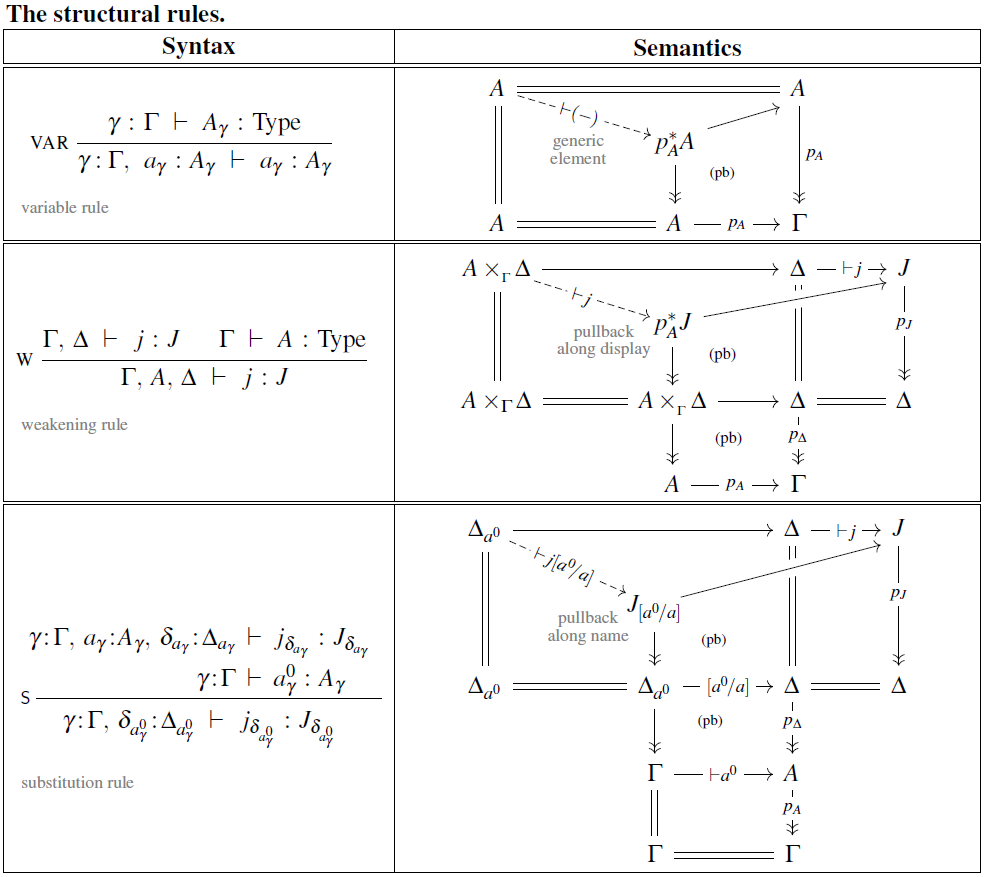Contents

# Contents

## Idea

In dependent type theory, what is known as the variable rule (or projection rule) is the structural inference rule which, informally speaking, asserts for any dependent type $A$ the apparent tautology that “given a variable $a \colon A$” (as a hypothesis/antecedent) “then we have that variable $a \colon A$” (as a succedent).

$VAR \frac{ \Gamma \;\;\;\; \vdash \;\;\;\; A \colon Type }{ \Gamma ,\; a \colon A \;\;\;\; \vdash \;\;\;\; a \colon A }$

As usual in dependent type theory, the meaning of this rule is a little less trivial than it may superficially seem by the generic type dependency involved: In the antecedent the variable may be picked out of a context telescope, and in the succedent it is implicitly weakened to depend on that full context, – which includes $a \colon A$ itself!

Accordingly, the categorical semantics as dependent types of the variable rule is given by the diagonal morphism on the object interpreting $A$, regarded as a section of the pullback of the bundle/display map $A \to \Gamma$ to its own total space: